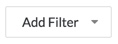# View a Field Production Report

## Objective

To view a Field Production Report.

## Background

Production quantities are a measurable amount of work on a construction project. For example, cubic yards of concrete, number of light fixtures, or linear feet of piping. In Procore, there are two types of production quantities:

• Budgeted production quantities refers to the measurable amount a team plans to install on a project.
• Installed production quantities refers to the actual amount installed on a project by a point in time.

The Field Production Report provides Procore users with the ability to compare a construction project's budgeted production quantities with its installed production quantities. With the appropriate permissions, you can view this report with the project's Reports or Timesheets tool.

## Things to Consider

• Required User Permissions:
• 'Read Only' permission or higher on the project's Reports tool.
AND
• 'Read Only' permission or higher on the project's Timesheets tool.
• Prerequisites:

## Steps

#### From the project's Reports tool

1. Navigate to the project's Reports tool.
2. Scroll to the Project Reports section.
3. Click Field Production Report.This reveals the Field Production Report.3. Click Add Filter to narrow the display results.4. Select one of the following:

• Cost Code
• Sub Job Name
• Unit Of Measure

The columns in the Field Production Report are as follows:

Hours:

• Budgeted (BH):  The number of Budgeted Hours you imported to Procore's project Admin tool.
• Actual (AH): The number of hours you have entered to date in Procore's labor tracking tools (Timesheets, Company Timecard, and Daily Log).
• Remaining (RH): The number of hours you have remaining from the hours you imported against the hours you have entered. Calculated as follows:
•  Budgeted Hours - Actual Hours = Remaining Hours
• Percent Used: The percent of hours used that you have imported against the hours you have entered. Calculated as follows:
• Actual Hours ÷ Budgeted Hours = Percent Used
• Projected At Completion: The number of hours needed to complete the process based on the Actual Production Rate (APR). Calculated as follows:
• (Remaining Quantities ÷ Actual Production Rate) + Actual Hours = Projected at Completion
• Earned Hours: The number of hours allowed to spend in order to stay on track with the Budgeted Production Rate (BPR). Calculated as follows:
• (Budgeted Hours x Actual Quantities) ÷ Budgeted Quantities = Earned Hours

Quantities:

• Budgeted (BQ): The number of Budgeted Quantities you imported to Procore's project Admin tool.
• Actual (AQ): The number of quantities you have entered to date in Procore's Timesheets tool.
• UoM: The unit of measure you imported with your budgeted quantities to Procore's Admin tool.
• Remaining: (RQ): The number of quantities you have remaining from the quantities you imported against the quantities you have entered. Calculated as follows:
• Budgeted Quantities - Actual Quantities = Remaining Quantities
• Percent Complete: The percent of quantities used that you have imported against the quantities you have entered. Calculated as follows:
• Actual Quantities ÷ Budgeted Quantities = Percent Complete

Production Rate:

• Budgeted (BPR): The number is the combination of the hours and quantities you have imported. Calculated as follows:
• Budgeted Quantities ÷ Budgeted Hours = Budgeted Production Rate (BPR)
• Actual (APR): The number is the combination of the hours and quantities you have entered. Calculated as follows:
• Actual Quantities ÷ Actual Hours = Actual Production Rate (APR)
• Variance: The number is the difference between your Budgeted Production Rate and Actual Production Rate. Calculated as follows:
• Actual Production Rate - Budgeted Production Rate = Variance

#### From the project's Timesheets tool

1. Navigate to the project's Timesheets tool.
2. Click Reports.
3. Click Field Production Report.This reveals the Field Production Report.3. Click Add Filter to narrow the display results.4. Select one of the following:

• Cost Code
• Sub Job Name
• Unit Of Measure

The columns in the Field Production Report are as follows:

Hours:

• Budgeted (BH):  The number of Budgeted Hours you imported to Procore's project Admin tool.
• Actual (AH): The number of hours you have entered to date in Procore's labor tracking tools (Timesheets, Company Timecard, and Daily Log).
• Remaining (RH): The number of hours you have remaining from the hours you imported against the hours you have entered. Calculated as follows:
•  Budgeted Hours - Actual Hours = Remaining Hours
• Percent Used: The percent of hours used that you have imported against the hours you have entered. Calculated as follows:
• Actual Hours ÷ Budgeted Hours = Percent Used
• Projected At Completion: The number of hours needed to complete the process based on the Actual Production Rate (APR). Calculated as follows:
• (Remaining Quantities ÷ Actual Production Rate) + Actual Hours = Projected at Completion
• Earned Hours: The number of hours allowed to spend in order to stay on track with the Budgeted Production Rate (BPR). Calculated as follows:
• (Budgeted Hours x Actual Quantities) ÷ Budgeted Quantities = Earned Hours

Quantities:

• Budgeted (BQ): The number of Budgeted Quantities you imported to Procore's project Admin tool.
• Actual (AQ): The number of quantities you have entered to date in Procore's Timesheets tool.
• UoM: The unit of measure you imported with your budgeted quantities to Procore's Admin tool.
• Remaining: (RQ): The number of quantities you have remaining from the quantities you imported against the quantities you have entered. Calculated as follows:
• Budgeted Quantities - Actual Quantities = Remaining Quantities
• Percent Complete: The percent of quantities used that you have imported against the quantities you have entered. Calculated as follows:
• Actual Quantities ÷ Budgeted Quantities = Percent Complete

Production Rate:

• Budgeted (BPR): The number is the combination of the hours and quantities you have imported. Calculated as follows:
• Budgeted Quantities ÷ Budgeted Hours = Budgeted Production Rate (BPR)
• Actual (APR): The number is the combination of the hours and quantities you have entered. Calculated as follows:
• Actual Quantities ÷ Actual Hours = Actual Production Rate (APR)
• Variance: The number is the difference between your Budgeted Production Rate and Actual Production Rate. Calculated as follows:
• Actual Production Rate - Budgeted Production Rate = Variance